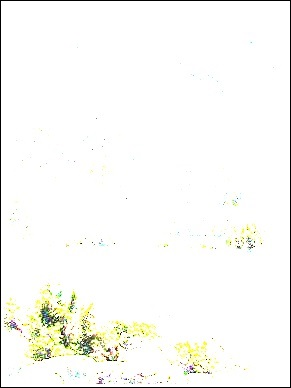# OpenCV - SQRBox Filter

You can perform the SQRBox Filter operation on an image using the boxFilter() method of the imgproc class. Following is the syntax of this method −

```sqrBoxFilter(src, dst, ddepth, ksize)
```

This method accepts the following parameters −

• src − A Mat object representing the source (input image) for this operation.

• dst − A Mat object representing the destination (output image) for this operation.

• ddepth − A variable of the type integer representing the depth of the output image.

• ksize − A Size object representing the size of the blurring kernel.

## Example

The following program demonstrates how to perform Sqrbox filter operation on a given image.

```import org.opencv.core.Core;
import org.opencv.core.Mat;
import org.opencv.core.Size;
import org.opencv.imgcodecs.Imgcodecs;
import org.opencv.imgproc.Imgproc;

public class SqrBoxFilterTest {
public static void main( String[] args ) {

// Reading the Image from the file and storing it in to a Matrix object
String file ="E:/OpenCV/chap11/filter_input.jpg";

// Creating an empty matrix to store the result
Mat dst = new Mat();

// Applying Box Filter effect on the Image
Imgproc.sqrBoxFilter(src, dst, -1, new Size(1, 1));

// Writing the image
Imgcodecs.imwrite("E:/OpenCV/chap11/sqrboxfilter.jpg", dst);

System.out.println("Image Processed");
}
}
```

Assume that following is the input image filter_input.jpg specified in the above program.## Output

On executing the program, you will get the following output −

```Image Processed
```

If you open the specified path, you can observe the output image as follows −